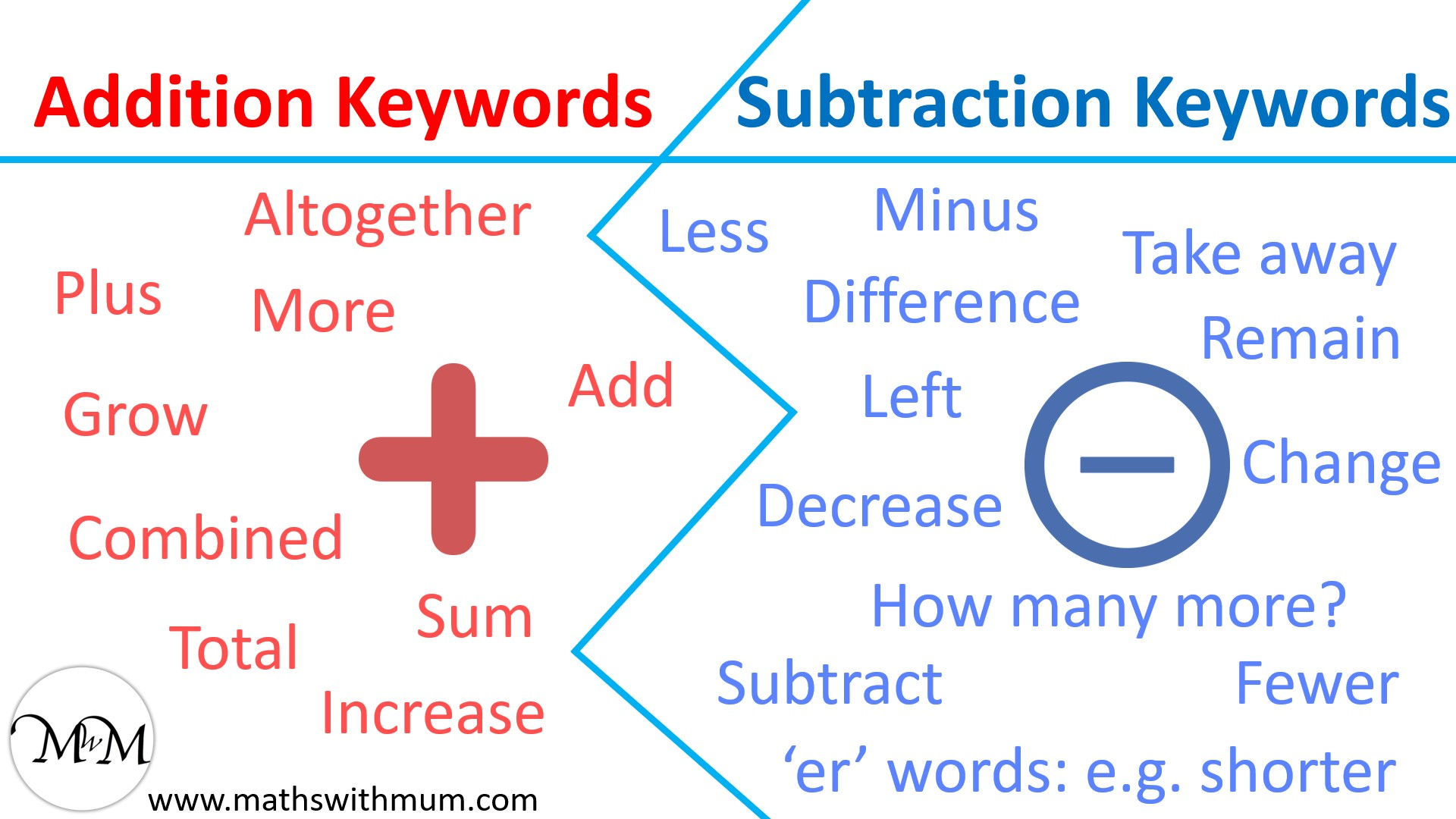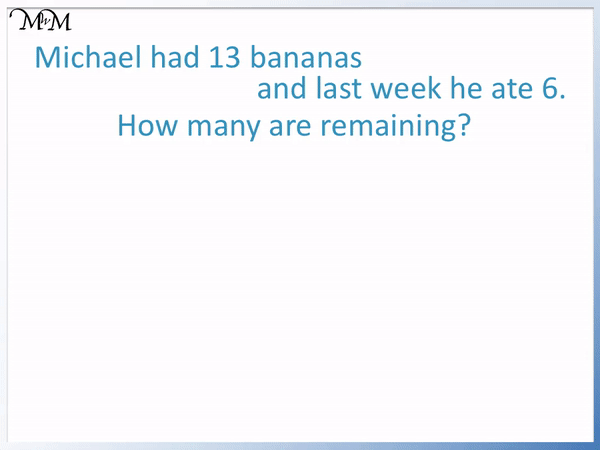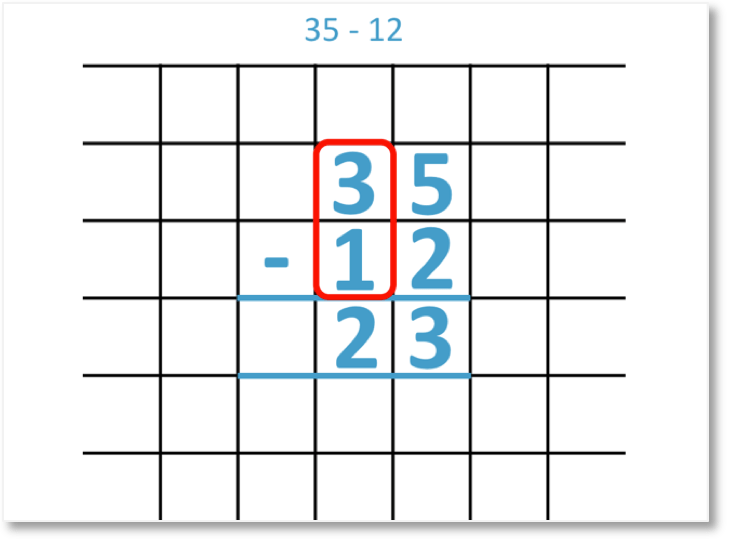# Number Facts Word Problems

Number Facts Word Problems• The word total tells us to add the two given numbers.
• If there are no keywords, look for the verb in the question to decide if we are adding or subtracting.
• 6 and 4 are number bonds to 10 and so, these numbers add to 10.
• Drawing a picture can help understand simple word problems.

Look for keywords that tell us to add or subtract.

If there are no keywords, use the verb to decide the context.• The numbers in this word problem are 10 and 5.
• There are none of the typical keywords in this question.
• Look at the verb in the question to decide whether we have an addition or a subtraction.
• If the verb means ‘combining’, then we have an addition.
• If the verb means ‘removing’ or ‘comparing’, then we have a subtraction.
• In this example, the verb is ‘gives’ and it means to remove.
• The word ‘left’ also implies that this is a subtraction.
• 10 – 5 = 5, we can use our number bonds to 10 to help us.

Verbs that mean ‘combining’ tell us to add.

Verbs that mean ‘removing’ or ‘comparing’ tell us to subtract.# Understanding Addition and Subtraction Word Problems with Number Facts

## How to Solve Addition and Subtraction Word Problems

To solve an addition or subtraction word problem, the first step is to decide whether it is an addition or subtraction operation.

It is important to read the entire worded problem when deciding if it is an addition or subtraction.

In general, if the numbers are being combined to make a total, then we have an addition word problem.

If the numbers are being removed, then we have a subtraction.

If the numbers are being compared, then we have a subtraction.

Keywords do not guarantee whether the word problem is an addition or subtraction, however they can be a big hint.

Here is a list of some common addition and subtraction keywords.Any of these keywords found in a word problem will most likely indicate whether it is an addition or subtraction.

Many word problems do not contain typical keywords. Look at the verbs in the word problem to decide if we are combining or removing the numbers.

## Examples of Word Problems

In the example below, “James has 6 apples and Jessica has 4 apples.”

We are asked, “How many do they have in total?”

In this example we have the addition keyword of ‘total’.

‘Total’ is a common addition keyword seen in worded problems. It gives us a clue that we are performing an addition. We just need to read the question carefully to decide if the two amounts are being combined.We are combining the two amounts of apples.

Drawing a picture can sometimes be a helpful way to visualise a word problem.

We will add the two numbers given in the word problem.

6 and 4 are number bonds to 10. It is helpful to look for common number facts.

6 + 4 = 10

There are 10 apples in total.

Here is another example of a worded problem.

“Olivia has 8 toys and Henry has 2 toys”, we are asked, “How many toys do they have in total?”

Again, we have the keyword ‘total’, which tells us to combine the two amounts.

We write down the two numbers involved in the worded problem and then add them.8 and 2 are another number bond to ten.

8 + 2 = 10

They have ten toys in total.

In this next example, “Sophia has 14 cherries and Alex has 5”. We are asked, “How many do they have altogether?”

Altogether is a common keyword we might see in word problems.

Altogether means to combine the values to make a total.

We read the full question again to check and we do need to combine the two values.

We have an addition word problem.Using our number facts to 10, we know that 4 + 5 = 9.

Therefore 14 + 5 = 19 because 14 is ten larger than 4.

Alternatively, we know that from our number bonds to 20, 15 + 5 = 20.

14 is one less than 15 and so, 14 + 5 is one less than 15 + 5.

14 + 5 is one less than 20, which gives us 19.

It can help to draw a picture of the word problem if you are struggling to understand it. It can also help to use manipulatives to act out the situation for young children.

Manipulatives are physical items that we can hold, such as wooden blocks, cubes and counters. We can use a counter or cube for each cherry in this question.

In this next example we have, “Chloe has 10 yoyos and gives 5 to her friend.” We are asked, “How many does she have left?”

There are none of the typical addition and subtraction keywords in this question.

In many word questions, we will not have familiar keywords to tell us what to do.

Instead, we look at the verb in the question. The verb is the action word or ‘doing word’.

If the verb implies that we are combining the amounts, then we may have an addition.

If the verb implies that we are removing an amount, then we may have a subtraction.

This is not a fixed rule because word problems can be written in different ways but it can be a big clue.The verb ‘gives’ implies that we are removing the 5 yoyos. Chloe is giving them away, or taking them away.

We have a subtraction word problem.

10 – 5 = 5 and so, there are 5 yoyos left.

The word ‘left’ also is a common word in word problems. It means what do we have after a subtraction has taken place and so, it gives us another clue that this is a subtraction.

The word ‘remaining’ is often seen instead of the word ‘left’ and it is a very similar clue.

Again, if using manipulatives, we could have started with 10 cubes and taken away 5.

In this word problem example we are told, “Michael has 13 bananas and last week he ate 6.” We are asked, “How many are remaining?”Again there are no typical keywords in this word problem so we look at the verb and try to understand the context.

The verb is ‘ate’. This implies that we remove some of the bananas by eating them. We are subtracting the 6 bananas from the original 13.

The word ‘remaining’ also implies that we have a subtraction. It means ‘what is left after doing a subtraction?’